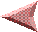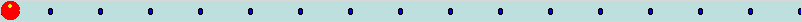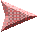PreviousMore loops and more secretsNext

You've already noticed that the `for` loop is a pretty useful command.

But, you can make it even more useful if you nest one loop command inside another.

When you nest loops, it means that the inner loop will run N times for each time the outer loop does a cycle.

Try typing this code into Komodo Edit and see what it does.

 ```for {set x 1} {\$x <= 5} {set x [expr \$x + 1]} { for {set y 1} {\$y <= 3} {set y [expr \$y + 1]} { tk_messageBox -type ok -message "X is \$x and Y is \$y" } } ```

You have to click the OK button a lot of times before you finish the two loops.

You can figure out how many times the innermost part of a loop will be executed. It will be the product of all the times you go through each loop. In the example above, that's 5 "X" loops and 3 "Y" loops. So there will be `3 x 5` passes for 15 tk_messageBox commands.

There's a couple of rules for using nested loops.

1. Each loop needs its own loop variable
2. Inner loops can't change the values of outer loop variables.

Now, in fact, neither of these rules is absolute, but if you break them, you better know just what you're doing and why.

For starters, lets look at the number guessing game where you use numbers from 0 to 100 and put 10 guesses on each row.

You can do that with a single loop, like the code just below this.

Dividing the contents of the variable `count` by 10 returns the row to put each button on. If you divide one whole number by another whole number in Tcl/Tk, it returns a whole number back to you. This is the quotient without any remainder or fractional part.

When `\$count` is less than 10, `\$count / 10` will be 0. When `\$count` is between 10 and 19, ```\$count / 10``` will be 1, and so forth.

The `expr` command's % operator divides one number by another and gives you the remainder instead of the quotient.

If you divide 23 by 10, the quotient is 2 with a remainder of 3. So `expr 23 %10` returns 3.

The remainder of `1 % 10` is `1`, the remainder of `12 % 10` is `2`, and so forth.

 `````` for {set count 0} {\$count < 100} {set count [expr \$count + 1]} { button .b_\$count -text \$count -command "tk_messageBox -type ok -message \$count" grid .b_\$count -row [expr \$count / 10] -column [expr \$count % 10] } ``````

This makes a display that looks like this:We can create the same buttons with a pair of nested loops: one loop for the rows and one for the columns.

 `````` set buttonNum 0 for {set row 1} {\$row <= 10} {set row [expr \$row + 1]} { for {set column 1} {\$column <= 10} {set column [expr \$column + 1]} { button .b_\$buttonNum -text "\$buttonNum" \ -command "tk_messageBox -type ok -message \$buttonNum" grid .b_\$buttonNum -row \$row -column \$column set buttonNum [expr \$buttonNum + 1] } } ``````

This is a little longer than the previous code, but it's also a little simpler. Being able to split the rows and columns into two spots means we don't need to think about the `%` operator any more.

But, it takes a lot of time to type in all of these `expr` commands to change the value of our loop variables.

Computer programmers don't like to waste a lot of time, so there are shorter ways to things that are very common. One very common thing for computer programs to do is to add a number to the value stored in a variable.

So, there's a command to increment a variable. It's called `incr`.

You use this command by telling `incr` what variable you want to add a value to, and the value to add. If you leave out the value to add, Tcl/Tk assumes you want to add 1. The value `1` is called a default. When you don't put some arguments onto a command line, you get a default value .

We can rewrite the previous loop using the `incr` command, and it will look like this:

 `````` set buttonNum 0 for {set row 1} {\$row <= 10} {incr row} { for {set column 1} {\$column <= 10} {incr column} { button .b_\$buttonNum -text "\$buttonNum" \ -command "tk_messageBox -type ok -message \$buttonNum" grid .b_\$buttonNum -row \$row -column \$column incr buttonNum } } ``````

It's not a big change, but it makes the program easier to read.

Using the nested loops makes it a little simpler to create the buttons on the game board.

When you've got 10 numbers in a row, the "Too High", and "Too Low" clues make sense. When the buttons are in a grid, those clues don't make so much sense. It would make more sense to say "LEFT", "RIGHT", "UP" or "DOWN". And then it's not a number guessing game, it's a position guessing game.

Instead of having a single secret number, we can change the game to have two secret numbers - a secret row and a secret column. When the player clicks too far to the left, they get told to go right, if they click to high, they get told to go down, etc.

It would be easy to write the loops like the next code. There's a problem with this. Look at it for a moment and see if you can see what the problem is. Here's a hint - the code will run just fine - it just won't do the right thing.

You might try typing it into Komodo Edit to see what it does.

 `````` for {set x 1} {\$x <= 10} {incr x} { for {set y 1} {\$y <= 10} {incr y} { if {\$x < \$secretX} { set message "Right" } if {\$x > \$secretX} { set message "Left" } if {\$y < \$secretY} { set message "Down" } if {\$y > \$secretY} { set message "Up" } button .b_\$buttonNum -text "Guess \$x \$y" \ -command "tk_messageBox -type ok -message \"\$message\"" grid .b_\$buttonNum -column \$x -row \$y incr buttonNum } } ``````

You probably noticed that when you select a position to the left and up, the code sets the `message` variable to "Left", and then overwrites that value and sets the `message` variable to "Up".

When you play the game, you need to go up and down until you find the correct row, then left and right to the correct column.

That's not a fun way to play the game. We can make it better.

Just like it's pretty common to want to add one value to the contents of a variable, it's common to want to add a new string onto the end of the contents of a variable.

The command to append one string onto the end of another is `append`.

Like the `incr` command, `append` command needs to know the variable to append new values to, and the values to append. Unlike `incr`, there is no default value for the `append` command.

To make a variable with the string `first second` in it, you might do something like this:

 ``````set message "first" append message " second" ``````

Notice that we've got a space after the first quote in the `append` command. The `append` command will append every single character that you give it to the string in your variable. It won't add any new characters (like a space) or skip any. If you left out the space, the new contents of `message` would be `firstsecond`

Here's the code for a position guessing game using the `tk_messageBox` commands. Notice the first `if` command. We'll discuss that right after you look at this example.

 ``````# Calculate 2 secret numbers between 1 and 10 set secretX [expr 1 + int(rand() * 10)] set secretY [expr 1 + int(rand() * 10)] # The variable buttonNum will make it easy to build # unique names for each button. set buttonNum 0 for {set x 1} {\$x <= 10} {incr x} { for {set y 1} {\$y <= 10} {incr y} { # Initialize the message to be an empty string set message {} # If the X and Y both match the secrets # the player has won. if {(\$x == \$secretX) && (\$y == \$secretY)} { set message "You Win" } # If x is less than secret, they player needs to guess # further to the right. if {\$x < \$secretX} { append message "Right " } # If x is greater than secret, they player needs to guess # further to the left. if {\$x > \$secretX} { append message "Left " } # If y is less than secret, they player needs to guess # further down. if {\$y < \$secretY} { append message "Down" } # If y is greater than secret, they player needs to guess # further up. if {\$y > \$secretY} { append message "Up" } button .b_\$buttonNum -text "Guess \$x \$y" \ -command "tk_messageBox -type ok -message {\$message}" grid .b_\$buttonNum -column \$x -row \$y incr buttonNum } } ``````

The `if` command in that example has two tests. One to check if `\$x` matchs `\$secretX` and one to check if `\$y` matchs `\$secretY`.

These tests are combined using the `&&` symbols. The `&&` means both of the tests have to be true for the `if` command to accept that the test is true.

The `&&` symbols are called boolean operators.

Boolean operators are just like the normal arithmetic operators you're familiar with like +, - and so forth.

But while arithmetic operators work with lots of numbers, the boolean operators only work with 2 values: TRUE and FALSE. We sometimes represent FALSE as a 0, and TRUE as any non-zero number, and sometimes as the words TRUE and FALSE, and sometimes we use the words yes for TRUE and no for FALSE.

The two boolean operators we use for building `if` command tests are:

 Symbol Name Example Description `&&` AND \$a && \$b Every argument must be true. `||` OR \$a || \$b At least one argument must be true.

You can group sets of boolean expressions Just like you can group parts of an arithmetic expression with parentheses.

For instance, if you want to make sure that the addition is done before the multipliction in an arithmetic expression, you'd write `(1 + 2) x 3`.

With boolean algebra, you group the tests in the order you want them done.

This test below is from the `if` command above. Tcl/Tk evaluates it in 3 steps:

``` (\$x == \$secretX) && (\$y == \$secretY) ```

First Tcl/Tk checks to see if `\$x` is the same as `\$secretX`. If it is, Tcl/Tk replaces that part of the command with a TRUE.

``` (TRUE) && (\$y == \$secretY) ```

If `\$x` is not the same as `\$secretX`, then we're done. A single FALSE means that the `&&` can't be TRUE.

But if `\$x` is the same as `\$secretX`, Tcl/Tk will check if `\$y` is the same as `\$secretY`, and then will replace that part of the command as necessary.

If `\$y` is the same as `\$secretY`, the new command will look like this:

``` (TRUE) && (TRUE) ```

Finally, Tcl/Tk compares the results to see if they are all TRUE. In this example, they are both TRUE, so the boolean expression is TRUE.

If both of these tests are TRUE, then the `if` command will look at the action associated with it.

Take another look at the `button` in the command above. The button text is `Guess \$x \$y`. The `\$x` and `\$y` will be replaced by the values of the x and y variables, so the actual text will be things like Guess 2 3.

I put the `\$x` and `\$y` into the button command so you can see how the buttons are arranged on the screen.

Do you need that text? What would happen if you had no `-text` argument for the `button` command, or if you just used a question mark, or your name? Try changing these on the button and see what happens.

Try playing with the nested loops in Komodo Edit. Change the program to be use a 15x15 grid instead of 10x10.

The important parts of this lesson are:

• You can nest one loop inside another.

• You can add a number to the contents of a variable with the `incr` command.

• You can use `incr` to add a negative number to the contents of a variable to do a subtractraction.

• You can make complex tests for if commands using boolean operators.

• You can append a string to a variable with the `append` command.

In the next lesson, we'll learn some more things about `snack` and make this program talk to us instead of using the `tk_messageBox` popups.PreviousNext